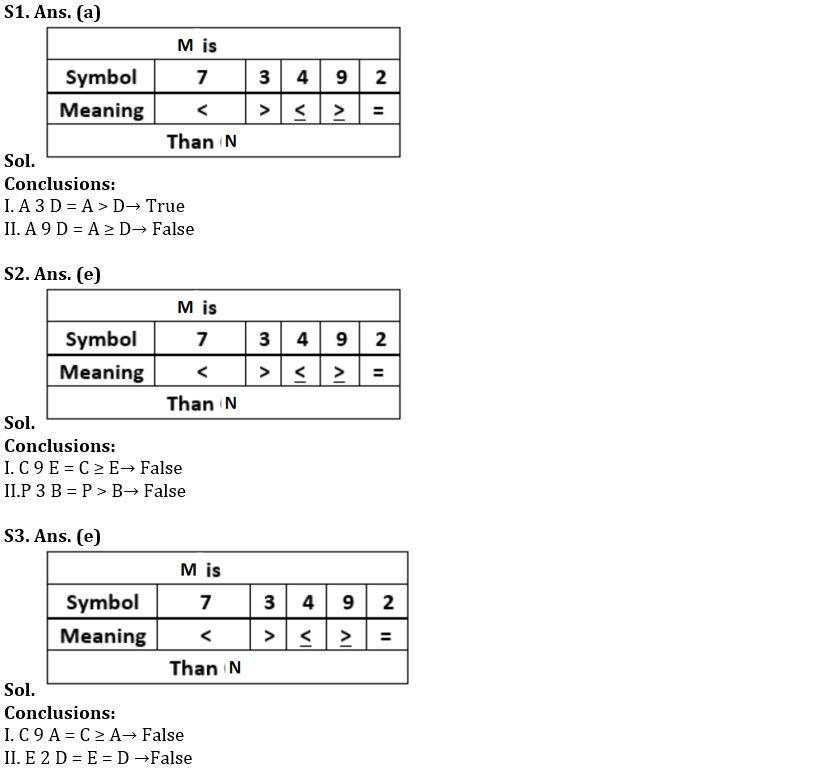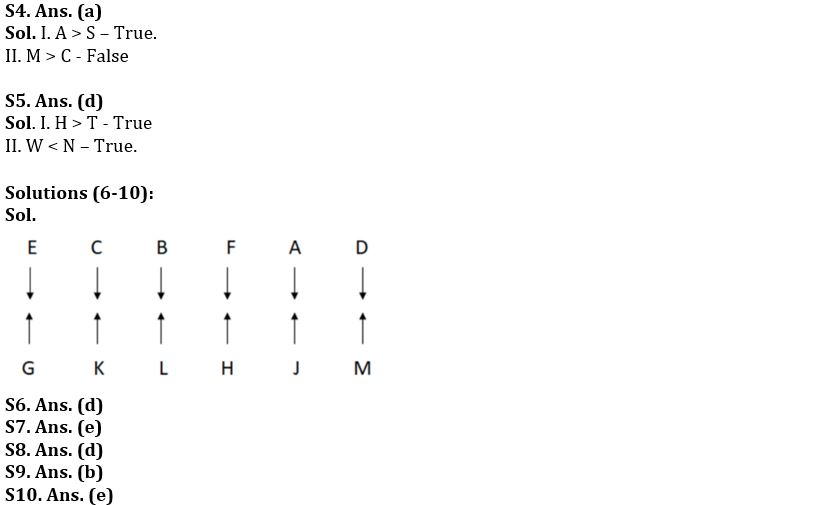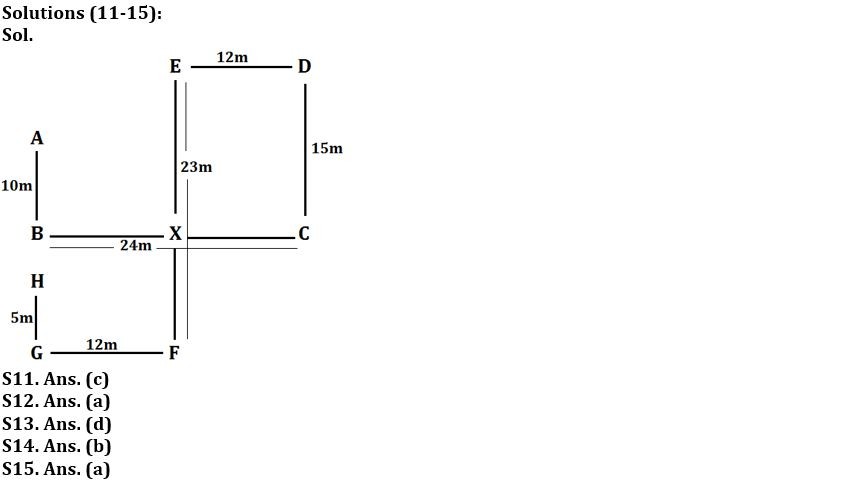Latest Banking jobs   »

# Reasoning Ability Quiz For IBPS SO Prelims 2022- 19th December

Direction (1-3): In the following questions, the symbols 4, 2, 3, 9, and 7 are used with the following meaning as illustrated below:
‘M 4 N’ means ‘M is either smaller than or equal to N’.
‘M 2 N’ means ‘M is neither greater than nor smaller than N’.
‘M 7 N’ means ‘M is neither greater than nor equal to N’.
‘M 3 N’ means ‘M is neither smaller than nor equal to N’
‘M 9 N’ means ‘M is either greater than or equal to N’.
Now in each of the following questions assuming the given statements to be true, find which of the conclusion(s) given below them is/are definitely true?

Q1. Statements: A 3 B 9 C 9 D 3 E, A 3 P 4 Q
Conclusions: I. A 3 D
II. A 9 D
(a) Only I is true
(b) Both I and II are true
(c) Either I or II is true
(d) Only II is true
(e) Neither I nor II is true

Q2. Statements: A 3 B 9 C 4 D 3 E, A 3 P 4 Q
Conclusions: I. C 9 E
II. P 3 B
(a) Only I is true
(b) Both I and II are true
(c) Either I or II is true
(d) Only II is true
(e) Neither I nor II is true

Q3. Statements: A 3 B 9 C 4 D 3 E, A 3 P 4 Q
Conclusions: I. C 9 A
II. E 2 D
(a) Only I is true
(b) Both I and II are true
(c) Either I or II is true
(d) Only II is true
(e) Neither I nor II is true

Direction (4-5): In the following question, some statements are followed by some conclusions. Assuming the given statements to be true, find which of the two conclusions follow(s) the given statements and choose appropriate answer choice.

Q4. Statements: S < R = C ≤ O = Y, T > O = F > N, A = K = T > M
Conclusions: I. A > S
II. M > C
(a) Only conclusion I is true
(b) Only conclusion II is true
(c) Either conclusion I or II is true
(d) Both conclusions I and II are true
(e) Neither conclusion I nor II is true

Q5. Statements: P ≤ U = H < N, P = W ≥ L > T, Q ≤ N = E
Conclusions: I. H > T
II. W < N
(a) Only conclusion I is true
(b) Only conclusion II is true
(c) Either conclusion I or II is true
(d) Both conclusions I and II are true
(e) Neither conclusion I nor II is true

Direction (6-10): Study the following data carefully and answer the questions accordingly.
Twelve people are sitting in two rows. In row 1 – A, B, C, D, E, and F are sitting and facing the south direction and in row 2 – G, H, J, K, L, and M are sitting and facing the north direction but not necessarily in the same order. M sits third to the right of the one who sits opposite B. E sits second to the right of B who is a neighbor of F. The one who sits opposite F, sits two places away from K. H sits to the right of K but not immediately. Less than three people sit between L and G. The one who sits opposite H, sits two places away from D who sits near A. G is not a neighbor of H.

Q6. Who sits second to the right of G?
(a) J
(b) The one who sits near to the one who sits opposite A.
(c) H
(d)The one, who sits opposite B
(e) None of these

Q7. How many people sit to the left of the one who sits opposite to L?
(a) One
(b) Two
(c) Four
(d) None
(e) Three

Q8. Who among the following are the immediate neighbors?
(a) E, B
(b) K, J
(c) M, H
(d) G, K
(e) None of these

Q9. How many persons sit between E and the one who sit opposite to M?
(a) One
(b) Four
(c) Three
(d) Two
(e) None

Q10. Four of the following five are same in a certain manner and forms a group, which among the following does not belong to the group?
(a) D, M
(b) C, K
(c) F, H
(d) E, G
(e) L, A

Direction (11-15): In the following questions, the symbols #, &, @ and \$ are used with the following meanings as illustrated below. Study the following information and answer the given questions:
Note: The directions which are given indicates exact directions.
P#Q – P is in the south direction of Q.
P@Q – P is in the north direction of Q.
P&Q – P is in the east direction of Q.
P\$Q – P is in the west direction of Q.
P£QS – P is the mid-point of QS vertically.

Point B is \$24m of point C. Point X£BC. Point E is @23m of point F. Point B is #10m of point A. Point D is &12m of point E. Point H is @5m of point G. Point G is \$12m of point F. Point C is #15m of point D.

Q11. What is the shortest distance between point A to Point X?
(a) 16m
(b) 10m
(c) 2√61m
(d) 5√13m
(e) None of these

Q12. What is the direction of point D with respect to point G?
(a) North-East
(b) South-West
(c) North-West
(d) East
(e) None of these

Q13. If Point M is @10m of point C, then what is the shortest distance and direction of point M with respect to point E?
(a) 13m, North-West
(b) 15m, North
(c) 10m, South-west
(d) 13m, South-east
(e) None of these

Q14. What is the direction of point F with respect to point C?
(a) North-East
(b) South-West
(c) North-West
(d) South
(e) None of these

Q15. What is the direction and shortest distance of point A with respect to point G?
(a) 18m, North
(b) 15m, North-east
(c) 13m, South
(d) 19m, South-west
(e) None of these

Solutions#### Congratulations!Union Budget 2023-24: Free PDF